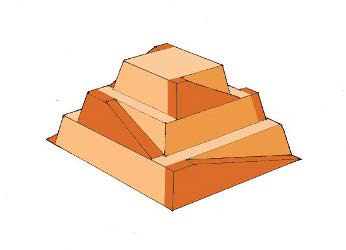×
Get Full Access to College Physics - 1 Edition - Chapter 7 - Problem 58pe
Get Full Access to College Physics - 1 Edition - Chapter 7 - Problem 58pe

×

# The awe-inspiring Great Pyramid of Cheops was built moreISBN: 9781938168000 42

## Solution for problem 58PE Chapter 7

College Physics | 1st Edition

• Textbook Solutions
• 2901 Step-by-step solutions solved by professors and subject experts
• Get 24/7 help from StudySoup virtual teaching assistantsCollege Physics | 1st Edition

4 5 1 409 Reviews
24
0
Problem 58PE

The awe-inspiring Great Pyramid of Cheops was built more than $$4500$$ years ago. Its square base, originally $$230 m$$ on a side, covered $$13.1$$ acres, and it was $$146 m$$ high, with a mass of about $$7 \times 10^{9} \mathrm{~kg}$$ . (The pyramid’s dimensions are slightly different today due to quarrying and some sagging.) Historians estimate that $$20,000$$ workers spent $$20$$ years to construct it, working $$12$$-hour days, $$330$$ days per year.

(a) Calculate the gravitational potential energy stored in the pyramid, given its center of mass is at one-fourth its height.

(b) Only a fraction of the workers lifted blocks; most were involved in support services such as building ramps (see Figure $$7.45$$), bringing food and water, and hauling blocks to the site. Calculate the efficiency of the workers who did the lifting, assuming there were $$1000$$ of them and they consumed food energy at the rate of $$300 \mathrm{kcal} / \mathrm{h}$$. What does your answer imply about how much of their work went into block-lifting, versus how much work went into friction and lifting and lowering their own bodies?

(c) Calculate the mass of food that had to be supplied each day, assuming that the average worker required $$3600 kcal$$ per day and that their diet was $$5 \%$$ protein, $$60 \%$$ carbohydrate, and $$35 \%$$ fat. (These proportions neglect the mass of bulk and nondigestible materials consumed.)Figure $$7.45$$ Ancient pyramids were probably constructed using ramps as simple machines. (credit: Franck Monnier, Wikimedia Commons)

Equation Transcription:Text Transcription:

4500

230 m

13.1

146 m

7 times 10^9 kg

20,000

20

12

330

7.45

1000

300 kcal/h

3600 kcal

5%

60%

35%

Step-by-Step Solution:

Solution 58PE :

a)

Step 1 of 4:-

In this section, we need to find the gravitational potential energy stored in the pyramid.

The mass of the pyramid is given as,.

The height of the pyramid is,.

The centre of mass of the pyramid lies at a point which is atof the height of the pyramid.

The gravitational potential energy stored in the pyramid can be calculated as,.

b)

Step 2 of 4

Step 3 of 4

##### ISBN: 9781938168000

Unlock Textbook Solution